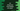# C# how to check if a HashTable is equal to another HashTable## C# program to check if a HashTable is equal to another HashTable:

To check if a HashTable is equal to another HashTable, we can use the Equals method defined in the HashTable class. This method takes another HashTable as the parameter and returns one boolean value defining both hash tables are equal or not.

So, to compare two HashTable in C#, it is not recommended to use == or =. In this post, we will learn how to use Equals method with an example.

### Definition of Equals:

Equals is defined as below:

``table1.Equals(table2)``

Here,

table1 and table2 are the hash tables we are comparing.

### Example of Equals:

Let’s take a look at the below example program:

``````using System;
using System.Collections;

public class Program {

public static void Main(string[] args) {
var firstTable = new Hashtable(){{1, "one"}, {2, "two"}, {3, "three"}, {4, "four"}};
var secondTable = new Hashtable(){{1, "one"}, {2, "two"}, {3, "three"}, {4, "four"}};
var thirdTable = new Hashtable(){{2, "one"}, {3, "two"}, {4, "three"}, {14, "four"}};
var fourthTable = firstTable;

Console.WriteLine(firstTable.Equals(secondTable));
Console.WriteLine(firstTable.Equals(thirdTable));
Console.WriteLine(firstTable.Equals(fourthTable));
Console.WriteLine(firstTable.Equals(firstTable));
}
}``````

It will print the below output:

``````False
False
True
True``````

### Explanation:

In this program:

• The first output is False because both firstTable and secondTable are different hash tables
• The second output is False because both firstTable and thirdTable are different hash tables.
• The third output is True because both firstTable and fourthTable are pointing to the same HashTable instance.
• The last output is True because Equals is checking firstTable with firstTable, because both are same.